Ex 10.2

Chapter 10 Class 12 Vector Algebra
Serial order wise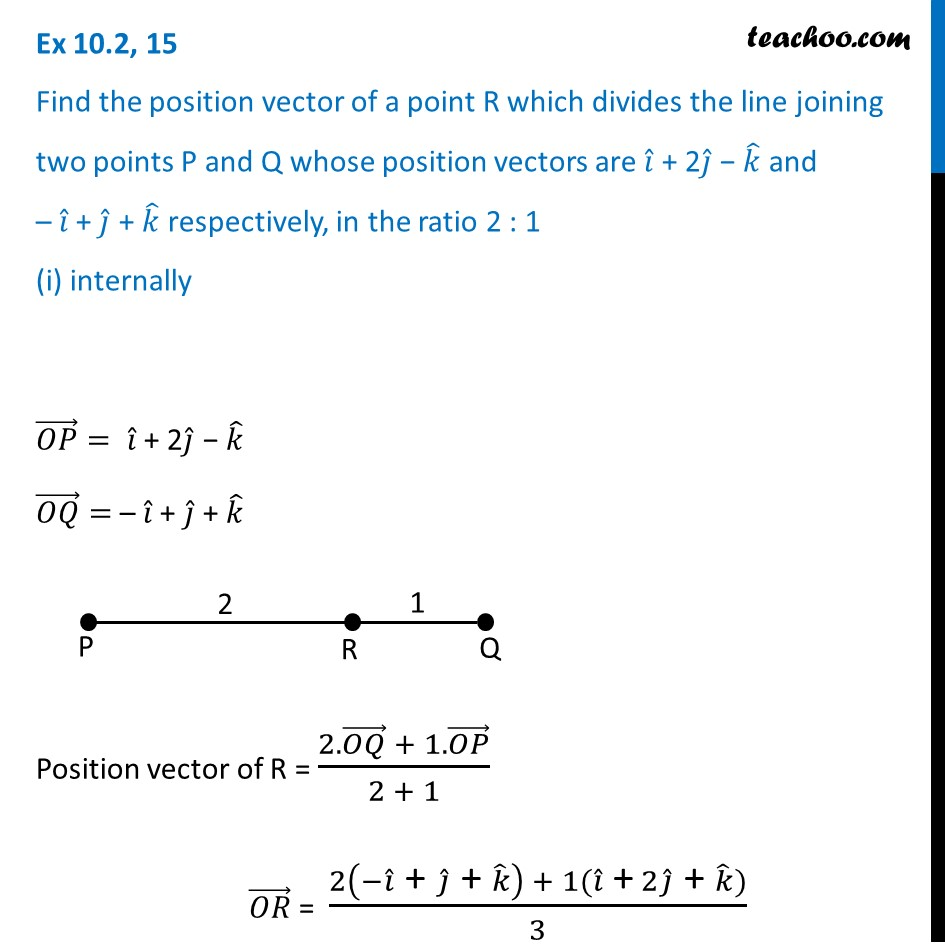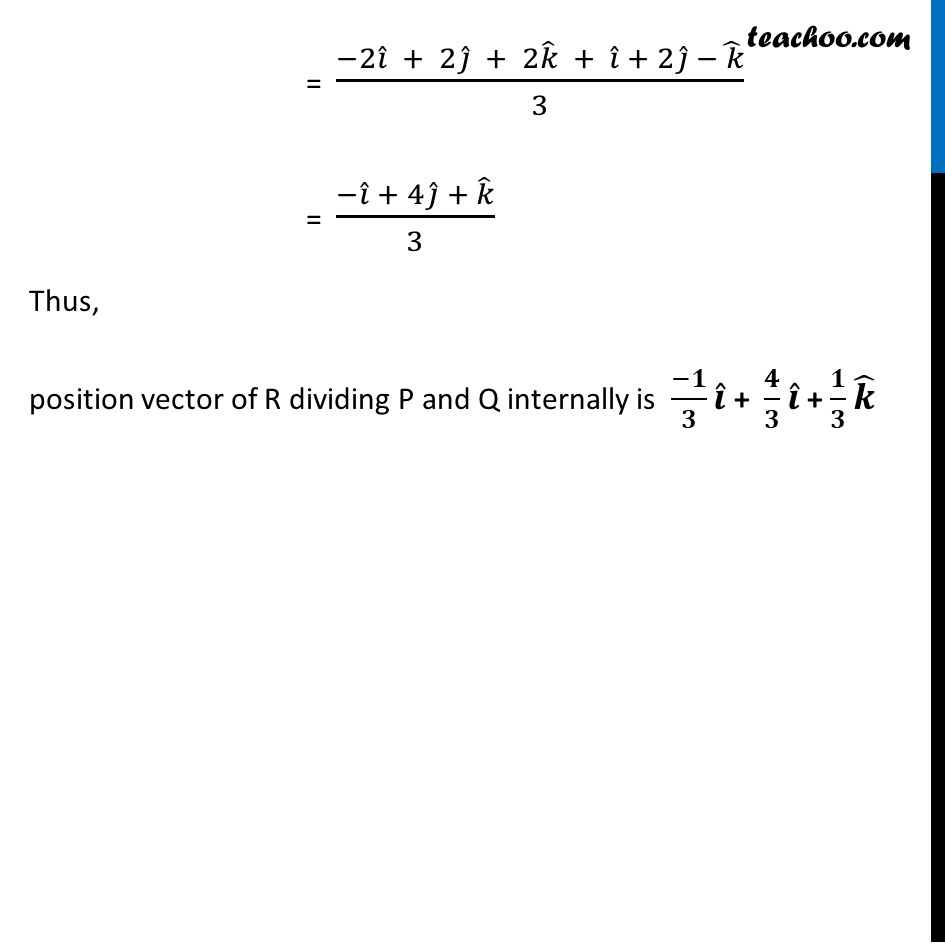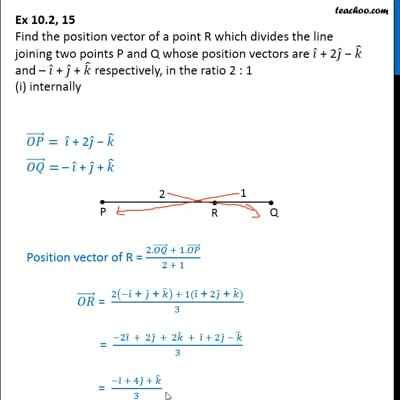This video is only available for Teachoo black users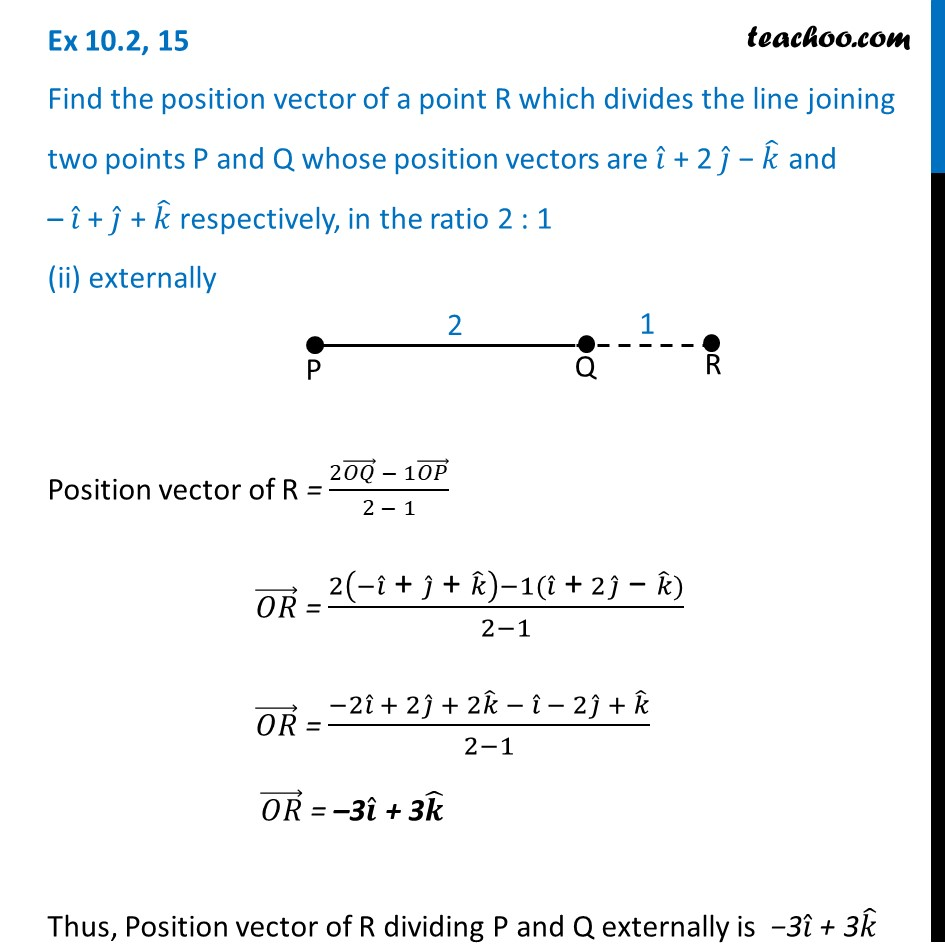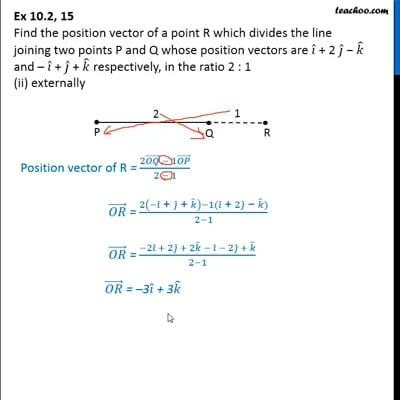This video is only available for Teachoo black users

Solve all your doubts with Teachoo Black (new monthly pack available now!)

### Transcript

Ex 10.2, 15 Find the position vector of a point R which divides the line joining two points P and Q whose position vectors are 𝑖 ̂ + 2𝑗 ̂ − 𝑘 ̂ and – 𝑖 ̂ + 𝑗 ̂ + 𝑘 ̂ respectively, in the ratio 2 : 1 (i) internally (𝑂𝑃) ⃗= 𝑖 ̂ + 2𝑗 ̂ − 𝑘 ̂ (𝑂𝑄) ⃗= – 𝑖 ̂ + 𝑗 ̂ + 𝑘 ̂ Position vector of R = (2.(𝑂𝑄) ⃗ + 1.(𝑂𝑃) ⃗)/(2 + 1) (𝑂𝑅) ⃗ = (2(−𝑖 ̂" + " 𝑗 ̂" + " 𝑘 ̂ ) + 1(𝑖 ̂" +" 2𝑗 ̂" + " 𝑘 ̂))/3 = (−2𝑖 ̂ + 2𝑗 ̂ + 2𝑘 ̂ + 𝑖 ̂ + 2𝑗 ̂ −( 𝑘) ̂)/3 = (−𝑖 ̂ + 4𝑗 ̂ + 𝑘 ̂)/3 Thus, position vector of R dividing P and Q internally is (−𝟏)/𝟑 𝒊 ̂ + 𝟒/𝟑 𝒊 ̂ + 𝟏/𝟑 𝒌 ̂ Ex 10.2, 15 Find the position vector of a point R which divides the line joining two points P and Q whose position vectors are 𝑖 ̂ + 2 𝑗 ̂ − 𝑘 ̂ and – 𝑖 ̂ + 𝑗 ̂ + 𝑘 ̂ respectively, in the ratio 2 : 1 (ii) externally Position vector of R = (2(𝑂𝑄) ⃗ − 1(𝑂𝑃) ⃗)/(2 − 1) (𝑂𝑅) ⃗ = (2(−𝑖 ̂" + " 𝑗 ̂" + " 𝑘 ̂ )−1(𝑖 ̂" + " 2𝑗 ̂" − " 𝑘 ̂))/(2−1) (𝑂𝑅) ⃗ = (−2𝑖 ̂ + 2𝑗 ̂ + 2𝑘 ̂ − 𝑖 ̂ − 2𝑗 ̂ + 𝑘 ̂)/(2−1) (𝑂𝑅) ⃗ = –3𝒊 ̂ + 3𝒌 ̂ Thus, Position vector of R dividing P and Q externally is −3𝑖 ̂ + 3𝑘 ̂